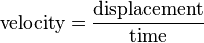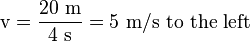# Velocity facts for kids

Kids Encyclopedia Facts
(Redirected from Constant velocity)

Velocity is a measure of how fast something moves in a particular direction. In other words, a velocity is a speed with a direction. For instance, if an object moves east at 7 metres per second (7 m/s), we would say that its velocity is 7 m/s to the east.

To calculate the velocity of an object, we divide its displacement (its change of position) by the time it took to change position.$\text{velocity} = \frac\text{displacement}\text{time}$

For example, if an object moves 20 meters (m) to the left in 4 seconds (s), its velocity (v) would be equal to:$\text{v} = \frac\text{20 m}\text{4 s} = \text{5 m/s to the left}$

A change in velocity is called an acceleration.fr:VitesseVelocity Facts for Kids. Kiddle Encyclopedia.